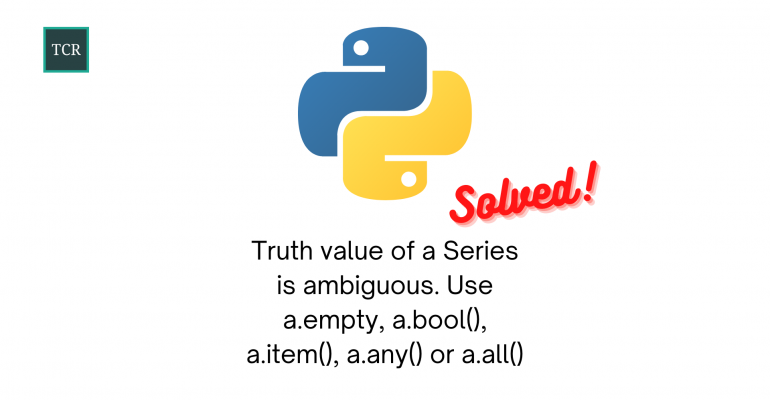Thanks to theidioms.com

### Infosys Tech Scholars Scholarship## [Solved] Truth value of a Series is ambiguous. Use a.empty, a.bool(), a.item(), a.any() or a.all()# [Solved] Truth value of a Series is ambiguous. Use a.empty, a.bool(), a.item(), a.any() or a.all()

Greetings! Some links on this site are affiliate links. That means that, if you choose to make a purchase, The Click Reader may earn a small commission at no extra cost to you. We greatly appreciate your support!

The Truth value of a Series is ambiguous. Use a.empty, a.bool(), a.item(), a.any() or a.all() error comes up when you are trying to chain conditional statements on a Pandas DataFrame using and/or operators.

Here are two cases when the error can come up:

``````# First Case
df[(df['col1'] > 20) and (df['col2'] <40)]

# Second Case
df[(df['col1'] > 20) or (df['col2'] <40)]``````

In both of the above two cases, the `or` and `and` Python conditional keywords require `truth-values`. However, in Pandas, these are considered ambiguous.

You can solve it by using bitwise operators such as | (or) or & (and) operators as follows:

``````# First Case
df[(df['col1'] > 20) & (df['col2'] <40)]

# Second Case
df[(df['col1'] > 20) | (df['col2'] <40)]``````

This will solve your Truth value of a Series is ambiguous. Use a.empty, a.bool(), a.item(), a.any() or a.all() error.Do you want to learn Python, Data Science, and Machine Learning while getting certified? Here are some best selling Datacamp courses that we recommend you enroll in:

1. Introduction to Python (Free Course) - 1,000,000+ students already enrolled!
2. Introduction to Data Science  in Python- 400,000+ students already enrolled!
3. Introduction to TensorFlow for Deep Learning with Python - 90,000+ students already enrolled!
4. Data Science and Machine Learning Bootcamp with R - 70,000+ students already enrolled!